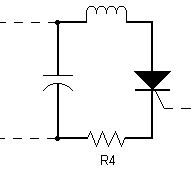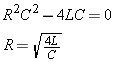# Damping Resistor

If the LCR circuit is critically damped, then no protection diode is needed. How do we choose the needed resistance?

## Damping ResistorAn under-damped circuit requires protection for electrolytic capacitors. A critically-damped circuit does not require protection, because the capacitor voltage will never become negative.

Critical damping produces the simplest possible circuit, and automatically works at any charge voltage. Whereas the V-switch’s extra capacitor must be adjusted whenever the voltage is changed.

This partial schematic shows how damping resistor R4 can be connected in series.

The damping resistor R4 also doubles as a current sensor.

The expected total circuit resistance can be found usingFor example, for C=12,000 µF and L=100 µH:Note that some resistance is provided by capacitor ESR (equivalent series resistance) and coil and connection resistance. For example, with an early coil used, the Java Inductor Simulator suggests that my coil has 0.06 ohms resistance.

The damping resistor R4 only supplies the portion of resistance to bring the total circuit to critical damping. This can only be found by testing with an oscilloscope to monitor the waveform.

 < Previous Page 11 of 37 Next >